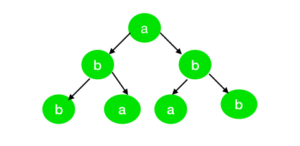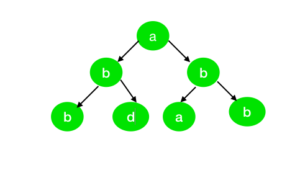# Check if Inorder traversal of a Binary Tree is palindrome or not

Given a binary tree and the task if to check if its Inorder Sequence is a palindrome or not.

Examples:

Input:Output: True
Explanation:
The Inorder sequence of the tree is “bbaaabb” which is a palindromic string.

Input:Output: False
Explanation:
The Inorder sequence of the tree is “bbdaabb” which is not a palindromic string.

## Recommended: Please try your approach on {IDE} first, before moving on to the solution.

Approach:
To solve the problem, refer to the following steps:

Below is the implementation of above approach:

## C++

 `// C++ Program to check if ` `// the Inorder sequence of a ` `// Binary Tree is a palindrome ` ` `  `#include ` `using` `namespace` `std; ` ` `  `// Define node of tree ` `struct` `node { ` `    ``char` `val; ` `    ``node* left; ` `    ``node* right; ` `}; ` ` `  `// Funtion to create the node ` `node* newnode(``char` `i) ` `{ ` `    ``node* temp = NULL; ` `    ``temp = ``new` `node(); ` `    ``temp->val = i; ` `    ``temp->left = NULL; ` `    ``temp->right = NULL; ` `    ``return` `temp; ` `} ` ` `  `// Function to convert the tree ` `// to the double linked list ` `node* conv_tree(node* root, ` `                ``node* shoot) ` `{ ` `    ``if` `(root->left != NULL) ` `        ``shoot = conv_tree(root->left, ` `                          ``shoot); ` ` `  `    ``root->left = shoot; ` ` `  `    ``if` `(shoot != NULL) ` `        ``shoot->right = root; ` ` `  `    ``shoot = root; ` ` `  `    ``if` `(root->right != NULL) ` `        ``shoot = conv_tree(root->right, ` `                          ``shoot); ` ` `  `    ``return` `shoot; ` `} ` ` `  `// Function for checking linked ` `// list is palindrom or not ` `int` `checkPalin(node* root) ` `{ ` `    ``node* voot = root; ` ` `  `    ``// Count the nodes ` `    ``// form the back ` `    ``int` `j = 0; ` ` `  `    ``// Set the pointer at the ` `    ``// very first node of the ` `    ``// linklist and count the ` `    ``// length of the linklist ` `    ``while` `(voot->left != NULL) { ` `        ``j = j + 1; ` `        ``voot = voot->left; ` `    ``} ` `    ``int` `i = 0; ` ` `  `    ``while` `(i < j) { ` `        ``// Check if the value matches ` `        ``if` `(voot->val != root->val) ` `            ``return` `0; ` ` `  `        ``else` `{ ` `            ``i = i + 1; ` `            ``j = j - 1; ` `            ``voot = voot->right; ` `            ``root = root->left; ` `        ``} ` `    ``} ` ` `  `    ``return` `1; ` `} ` ` `  `// Driver Program ` `int` `main() ` `{ ` `    ``node* root = newnode(``'b'``); ` `    ``root->left = newnode(``'b'``); ` `    ``root->right = newnode(``'a'``); ` `    ``root->left->right = newnode(``'b'``); ` `    ``root->left->left = newnode(``'a'``); ` ` `  `    ``node* shoot = conv_tree(root, NULL); ` ` `  `    ``if` `(checkPalin(shoot)) ` `        ``cout << ``"True"` `<< endl; ` `    ``else` `        ``cout << ``"False"` `<< endl; ` `} `

## Java

 `// Java Program to check if ` `// the Inorder sequence of a ` `// Binary Tree is a palindrome ` `import` `java.util.*; ` `class` `GFG{ ` ` `  `// Define node of tree ` `static` `class` `node ` `{ ` `    ``char` `val; ` `    ``node left; ` `    ``node right; ` `}; ` ` `  `// Funtion to create the node ` `static` `node newnode(``char` `i) ` `{ ` `    ``node temp = ``null``; ` `    ``temp = ``new` `node(); ` `    ``temp.val = i; ` `    ``temp.left = ``null``; ` `    ``temp.right = ``null``; ` `    ``return` `temp; ` `} ` ` `  `// Function to convert the tree ` `// to the double linked list ` `static` `node conv_tree(node root, ` `                      ``node shoot) ` `{ ` `    ``if` `(root.left != ``null``) ` `        ``shoot = conv_tree(root.left, ` `                          ``shoot); ` ` `  `    ``root.left = shoot; ` ` `  `    ``if` `(shoot != ``null``) ` `        ``shoot.right = root; ` ` `  `    ``shoot = root; ` ` `  `    ``if` `(root.right != ``null``) ` `        ``shoot = conv_tree(root.right, ` `                          ``shoot); ` ` `  `    ``return` `shoot; ` `} ` ` `  `// Function for checking linked ` `// list is palindrom or not ` `static` `int` `checkPalin(node root) ` `{ ` `    ``node voot = root; ` ` `  `    ``// Count the nodes ` `    ``// form the back ` `    ``int` `j = ``0``; ` ` `  `    ``// Set the pointer at the ` `    ``// very first node of the ` `    ``// linklist and count the ` `    ``// length of the linklist ` `    ``while` `(voot.left != ``null``) ` `    ``{ ` `        ``j = j + ``1``; ` `        ``voot = voot.left; ` `    ``} ` `    ``int` `i = ``0``; ` ` `  `    ``while` `(i < j)  ` `    ``{ ` `        ``// Check if the value matches ` `        ``if` `(voot.val != root.val) ` `            ``return` `0``; ` ` `  `        ``else`  `        ``{ ` `            ``i = i + ``1``; ` `            ``j = j - ``1``; ` `            ``voot = voot.right; ` `            ``root = root.left; ` `        ``} ` `    ``} ` `    ``return` `1``; ` `} ` ` `  `// Driver Code ` `public` `static` `void` `main(String[] args) ` `{ ` `    ``node root = newnode(``'b'``); ` `    ``root.left = newnode(``'b'``); ` `    ``root.right = newnode(``'a'``); ` `    ``root.left.right = newnode(``'b'``); ` `    ``root.left.left = newnode(``'a'``); ` ` `  `    ``node shoot = conv_tree(root, ``null``); ` ` `  `    ``if` `(checkPalin(shoot) == ``1``) ` `        ``System.out.print(``"True"` `+ ``"\n"``); ` `    ``else` `        ``System.out.print(``"False"` `+ ``"\n"``); ` `} ` `} ` ` `  `// This code is contributed by 29AjayKumar `

## Python3

 `# Python3 program to check if ` `# the Inorder sequence of a ` `# Binary Tree is a palindrome ` ` `  `# Define node of tree ` `class` `Node:  ` `    ``def` `__init__(``self``,val):  ` `         `  `        ``self``.val ``=` `val  ` `        ``self``.left ``=` `None` `        ``self``.right ``=` `None` ` `  `# Funtion to create the node ` `def` `newnode(i): ` ` `  `    ``#temp = None; ` `    ``temp ``=` `Node(i); ` `    ``temp.val ``=` `i; ` `    ``temp.left ``=` `None``; ` `    ``temp.right ``=` `None``; ` `    ``return` `temp; ` ` `  `# Function to convert the tree ` `# to the double linked list ` `def` `conv_tree(root, shoot): ` ` `  `    ``if` `(root.left !``=` `None``): ` `        ``shoot ``=` `conv_tree(root.left, shoot); ` ` `  `    ``root.left ``=` `shoot; ` ` `  `    ``if` `(shoot !``=` `None``): ` `        ``shoot.right ``=` `root; ` ` `  `    ``shoot ``=` `root; ` ` `  `    ``if` `(root.right !``=` `None``): ` `        ``shoot ``=` `conv_tree(root.right, shoot); ` ` `  `    ``return` `shoot; ` ` `  `# Function for checking linked ` `# list is palindrom or not ` `def` `checkPalin(root): ` ` `  `    ``voot ``=` `root; ` ` `  `    ``# Count the nodes ` `    ``# form the back ` `    ``j ``=` `0``; ` ` `  `    ``# Set the pointer at the ` `    ``# very first node of the ` `    ``# linklist and count the ` `    ``# length of the linklist ` `    ``while` `(voot.left !``=` `None``):  ` `        ``j ``=` `j ``+` `1``; ` `        ``voot ``=` `voot.left; ` `     `  `    ``i ``=` `0``; ` ` `  `    ``while` `(i < j): ` `         `  `        ``# Check if the value matches ` `        ``if` `(voot.val !``=` `root.val): ` `            ``return` `0``; ` `        ``else``: ` `            ``i ``=` `i ``+` `1``; ` `            ``j ``=` `j ``-` `1``; ` `            ``voot ``=` `voot.right; ` `            ``root ``=` `root.left; ` `             `  `    ``return` `1``; ` ` `  `# Driver code ` `if` `__name__``=``=``'__main__'``:  ` ` `  `    ``root ``=` `newnode(``'b'``); ` `    ``root.left ``=` `newnode(``'b'``); ` `    ``root.right ``=` `newnode(``'a'``); ` `    ``root.left.right ``=` `newnode(``'b'``); ` `    ``root.left.left ``=` `newnode(``'a'``); ` ` `  `    ``shoot ``=` `conv_tree(root, ``None``); ` ` `  `    ``if` `(checkPalin(shoot)): ` `        ``print``(``"True"``); ` `    ``else``: ` `        ``print``(``"False"``); ` ` `  `# This code is contributed by AbhiThakur `

## C#

 `// C# program to check if the inorder   ` `// sequence of a Binary Tree is a  ` `// palindrome ` `using` `System; ` ` `  `class` `GFG{ ` ` `  `// Define node of tree ` `class` `node ` `{ ` `    ``public` `char` `val; ` `    ``public` `node left; ` `    ``public` `node right; ` `}; ` ` `  `// Funtion to create the node ` `static` `node newnode(``char` `i) ` `{ ` `    ``node temp = ``null``; ` `    ``temp = ``new` `node(); ` `    ``temp.val = i; ` `    ``temp.left = ``null``; ` `    ``temp.right = ``null``; ` ` `  `    ``return` `temp; ` `} ` ` `  `// Function to convert the tree ` `// to the double linked list ` `static` `node conv_tree(node root, ` `                      ``node shoot) ` `{ ` `    ``if` `(root.left != ``null``) ` `        ``shoot = conv_tree(root.left, ` `                          ``shoot); ` ` `  `    ``root.left = shoot; ` ` `  `    ``if` `(shoot != ``null``) ` `        ``shoot.right = root; ` ` `  `    ``shoot = root; ` ` `  `    ``if` `(root.right != ``null``) ` `        ``shoot = conv_tree(root.right, ` `                          ``shoot); ` ` `  `    ``return` `shoot; ` `} ` ` `  `// Function for checking linked ` `// list is palindrom or not ` `static` `int` `checkPalin(node root) ` `{ ` `    ``node voot = root; ` ` `  `    ``// Count the nodes ` `    ``// form the back ` `    ``int` `j = 0; ` ` `  `    ``// Set the pointer at the ` `    ``// very first node of the ` `    ``// linklist and count the ` `    ``// length of the linklist ` `    ``while` `(voot.left != ``null``) ` `    ``{ ` `        ``j = j + 1; ` `        ``voot = voot.left; ` `    ``} ` `    ``int` `i = 0; ` ` `  `    ``while` `(i < j)  ` `    ``{ ` `         `  `        ``// Check if the value matches ` `        ``if` `(voot.val != root.val) ` `            ``return` `0; ` `        ``else` `        ``{ ` `            ``i = i + 1; ` `            ``j = j - 1; ` `            ``voot = voot.right; ` `            ``root = root.left; ` `        ``} ` `    ``} ` `    ``return` `1; ` `} ` ` `  `// Driver Code ` `public` `static` `void` `Main(String[] args) ` `{ ` `    ``node root = newnode(``'b'``); ` `    ``root.left = newnode(``'b'``); ` `    ``root.right = newnode(``'a'``); ` `    ``root.left.right = newnode(``'b'``); ` `    ``root.left.left = newnode(``'a'``); ` ` `  `    ``node shoot = conv_tree(root, ``null``); ` ` `  `    ``if` `(checkPalin(shoot) == 1) ` `        ``Console.Write(``"True"` `+ ``"\n"``); ` `    ``else` `        ``Console.Write(``"False"` `+ ``"\n"``); ` `} ` `} ` ` `  `// This code is contributed by 29AjayKumar `

Output:

```True
```

Time Complexity: O(N)
Auxiliary Space: O(1)

Attention reader! Don’t stop learning now. Get hold of all the important DSA concepts with the DSA Self Paced Course at a student-friendly price and become industry ready.

My Personal Notes arrow_drop_upCheck out this Author's contributed articles.

If you like GeeksforGeeks and would like to contribute, you can also write an article using contribute.geeksforgeeks.org or mail your article to contribute@geeksforgeeks.org. See your article appearing on the GeeksforGeeks main page and help other Geeks.

Please Improve this article if you find anything incorrect by clicking on the "Improve Article" button below.